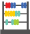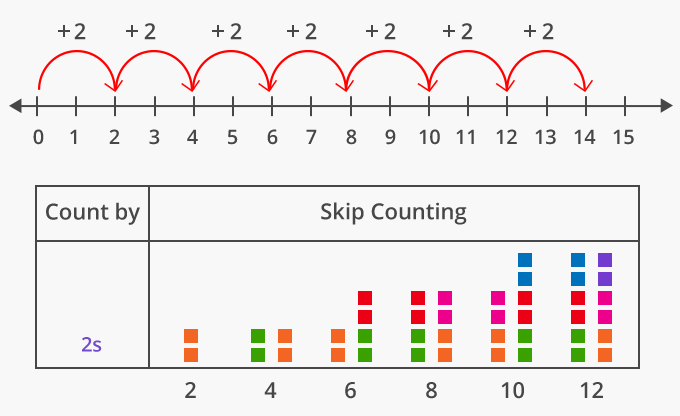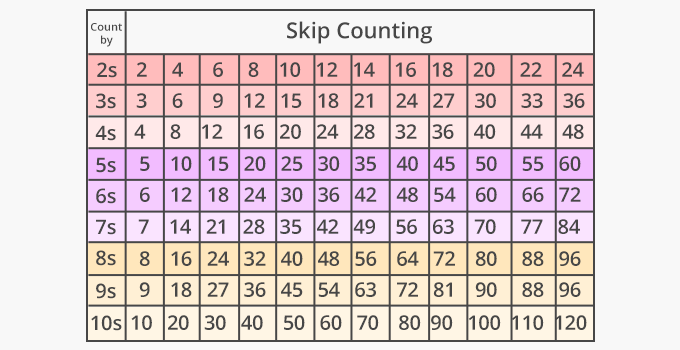# Skip Count - Definition with Examples

The Complete K-5 Math Learning Program Built for Your Child

• 30 Million Kids

Loved by kids and parent worldwide

• 50,000 Schools

Trusted by teachers across schools

• Comprehensive Curriculum

Aligned to Common Core

##Let's learn!

What is skip counting?
In math, skip counting can be defined as the method of counting forward by numbers other than 1.
To skip count, we keep adding the same number each time to the previous number.

Here, we are skip counting by 2 on a number line. So, starting at 0, the next number will be 0 + 2 = 2, then, 2 + 2 = 4, then 4 + 2 = 6, then 6 + 2 = 8, and then, 10, 12, 14, 16, 18 and so on.We can skip count by any number. Here’s how skip counting by numbers 2 to 10, begin to form patterns, starting at 0.Fun Facts Knowing how to skip count helps ones to remember math multiplication tables and facts.

##Let's sing!

Skip counting can be so much fun,
Jump the same number from the previous one.
A pattern you will see , when you are done!

##Let's do it!

After your child has solved worksheets on skip counting, show them how skip counting can help them to multiply numbers.
Further, you can give them a set of numbers (jumbled consecutive multiples of a number), ask them arrange the numbers in order, from least to greatest and observe the pattern. Then, ask them to by which number have they skip counted.

##Related math vocabulary

Won Numerous Awards & Honors